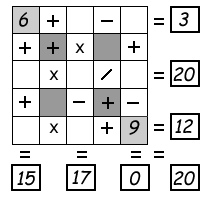Select PagePrintable version

Each row, column & diagonal is an equation and you use the numbers 1 to 9 to complete the equations.   Each number can be used only once. Some numbers have been provided to get you started. Find the remaining numbers to satisfy all of the resulting equations.

Not sure where to start?  Have a look at the example walk-through below.NOTE – These puzzles are supplied in 4 levels of difficulty, depending on how many numbers are supplied – 0, 1, 2 or 3 numbers. The easiest level is Equate+3, and the hardest is Equate+0. The puzzle above is an Equate+2.

Remember the order in which you should carry out arithmetical calculations!

Use BODMAS:

Brackets are calculated first
Then Orders (Powers and Square Roots)
Then Division or Multiplication
Finally Addition or Subtraction

This also can be remembered as PEMDAS:

Parentheses are calculated first
Then Exponents (Powers and Square Roots)
Then Multiplication or Division
Finally Addition or Subtraction

### Solution### Example walkthough

The Puzzle

This puzzle is an example of an “Equate+2” puzzle, where 2 of the 9 unknowns are provided. Letters (B, C, D, E, F, G and H) have been inserted in the original puzzle example, so that the unknown values can be determined.The Solution

We can write the following 7 equations for this puzzle.The equations and unknown values can be solved as follows:As we go, we can eliminate and confirm the possible solutions into this table:Giving us the final solution:## Feedback

There are more than one way of doing these puzzles and may well be more than one answer.  Please let me and others know what alternatives you find by commenting below.  We also welcome general comments on the subject and any feedback you’d like to give.   If you have a question that need a response from me or you would like to contact me privately, please use the contact form.

## Get more puzzles!

If you’ve enjoyed doing the puzzles, consider ordering the book; 150+ of the best puzzles in a handy pocket sized format. Click here for full details.

### Share This

Share this post with your friends!